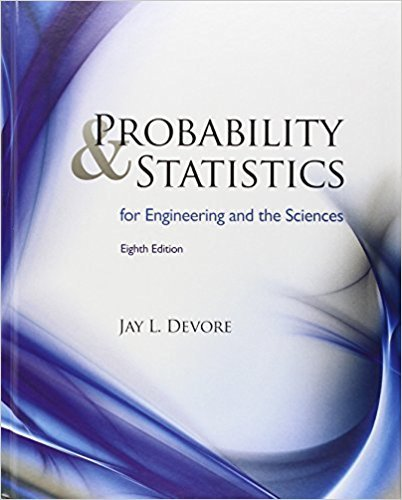### Solution Found!# The article “Oxygen Consumption During Fire Suppression:

Chapter 1, Problem 44E

(choose chapter or problem)

Get Unlimited Answers! Check out our subscriptions
QUESTION:

Problem 44E

The article “Oxygen Consumption During Fire Suppression: Error of Heart Rate Estimation” (Ergonomics, 1991: 1469–1474) reported the following data on oxygen consumption (mL/kg/min) for a sample of ten firefighters performing a fire-suppression simulation:Compute the following:

a. The sample range

b. The sample variance s2 from the definition (i.e., by first computing deviations, then squaring them, etc.)

c. The sample standard deviation

d. s2 using the shortcut method

QUESTION:

Problem 44E

The article “Oxygen Consumption During Fire Suppression: Error of Heart Rate Estimation” (Ergonomics, 1991: 1469–1474) reported the following data on oxygen consumption (mL/kg/min) for a sample of ten firefighters performing a fire-suppression simulation:Compute the following:

a. The sample range

b. The sample variance s2 from the definition (i.e., by first computing deviations, then squaring them, etc.)

c. The sample standard deviation

d. s2 using the shortcut method

Solution Step 1 of 4 a) We have to compute the sample range The given data is 29.5, 49.3, 30.6, 28.2, 28.0, 26.3, 33.9, 29.4, 23.5, 31.6 Range = Largest value - smallest value = 49.3 - 23.5 = 25.8 Hence Range is 25.8